# Anti-seismic Effect of the Shock Absorption Layer in Tunnel

Anti-seismic Effect of the Shock Absorption Layer in Tunnel
Key Laboratory of Road & Bridges and Underground Engineering of Gansu Province, Lanzhou Jiaotong University, Lanzhou 730070, China

National and Provincial Joint Engineering Laboratory of Road & Bridge Disaster Prevention and Control, Lanzhou Jiaotong University, Lanzhou 730070, China

Corresponding Author Email:
caoxp@mail.lzjtu.cn
Page:
41-47
|
DOI:
https://doi.org/10.18280/ijsse.100106
20 May 2019
|
Accepted:
15 November 2019
|
Published:
29 February 2020
| Citation

OPEN ACCESS

Abstract:

Tunnels play a vital role in the transportation industry and many other industries. In fact, a tunnel damaged by an earthquake has a serious impact on traffic. Therefore, tunnel destruction induced by earthquakes have been paid more and more attentions. In this study, vibration theory is used for a simplified mechanical model of a tunnel lining system composed of a primary lining, a layer of shock absorption and a second lining. The parameters, such as the mass ratio, the damping ratio and the stiffness ratio of the vibration equation, are simplified correspondingly and solved in the complex domain, resulting in a simplified transfer coefficient. At the same time, numerical simulation and analysis are also carried out. The results show that: 1) The shock absorption layer with a low stiffness ratio has a good damping effect under low-frequency vibration, while under high-frequency vibration, the stiffness ratio has less influence on the transmission coefficient. 2) By analyzing the results of the theoretical calculation and numerical simulation, the tunnel structure under high-frequency vibration can be seen, and when the shock absorption layer stiffness is in close proximity to the second lining stiffness, the deformation and stress of the tunnel lining are the smallest. In general, the application of double-lining in strong earthquake zones can reduce the vibration of the tunnel lining and protect the tunnel from damage.

Keywords:

tunnel, anti-seismic and shock, theory of vibration, finite element method, stiffness ratio

1. Introduction

With the rapid development of the economy and society in China, tunnels have to be built in areas of high earthquake-intensity where earthquakes frequently occur. Many investigations conducted after earthquakes have occurred indicate that the damage caused to the tunnel lining by a massive earthquake is very serious [1-5], and there are various forms of damage. Anti-seismic design for underground structures mainly concentrates on the following aspects. First, the input direction of the seismic wave is important, which would avoid the input direction of the seismic wave perpendicular to the longitudinal direction of the tunnel. However, to construct a tunnel to avoid the direction of the most harmful seismic waves is impossible because of the random behaviour of earthquakes. Second, the tunnel lining and the surrounding rock are reinforced to increase their strength and stiffness in order to resist the destruction of earthquake effects. Third, a layer of shock absorption is set between the tunnel lining and the surrounding rock to absorb most of the seismic energy to prevent damage to the tunnel through shock absorption.

Over the years, the problems of tunnel construction have been studied by many researchers, and many studies have been published [5-9]. Okamoto and Choshiro  pointed out that great developments have been made through research regarding the numerical analysis of the tunnel dynamic response at home and abroad.

Much of the research is based on a comprehensive analysis of the results and is obtained from a model test and numerical calculation by the Finite Element Method. To date, laboratory model tests [11-14] as well as some numerical simulations [15, 16] have been conducted in many previous studies to investigate the seismic response of tunnels; many valuable achievements have been gained from these research methods. Furthermore, Wang and Cui  established a damping model by setting a shock absorption layer in the support system of a tunnel; the shock absorption layer stiffness and the input of the frequency of the seismic and shock absorption layer damping on the damping effect of the tunnel were researched. The results provided good references for the aseismic design of tunnel structures in a highly seismic area.

In addition, the motion of an earthquake is a non-stationary random process; thus, random vibration theory was developed in the 1950s based on the probability of a structure with random vibration theory. So far, the linear system of the stationary random vibration problem research is the most developed; it is also the most widely used in engineering and gradually has become a classic theory. In the early 1960s, the study of nonlinear random vibration problems developed some more effective methods of analysis. However, Cao  pointed out that the random vibration of nonlinear systems and a linear system under non-stationary random vibration excitation of a random vibration problem are still in development. In recent years, a great deal of progress has been made [19, 20] for analysis of the seismic dynamic response of underground structures by using the random vibration theory. Yan et al.  Simulated the lateral and overall random seismic responses of underground structures by taking the approach of impulse response function of a random vibration.

Much of the literature is about tunnel anti-seismic and shock designs; the model test and numerical simulation method are used widely, but relevant studies based on vibration theory are notably limited. In this paper, the vibration theory is used for a simplified mechanical model of the tunnel lining system composed of the primary lining, a layer of shock absorption and a second lining. To obtain the optimum stiffness ratio, the present paper attempts to make a systematic analysis according to the mass ratio, damping ratio and stiffness ratio. The outline of this paper is as follows. In Sections 2–4, the theoretical formulations for a simplified mechanical model of the tunnel lining system are given, and the formula is derived and calculated theoretically. In Section 5, the numerical model of a tunnel lining structure system with a shock absorption layer is established, and the calculation results are discussed. The conclusions are summarized in Section 6.

2. The Tunnel Damping Mechanics Model and the Vibration Equation

To study these variables easily, the surrounding rock, the shock absorption layer and the lining are considered to be three particles (Figure 1). It is assumed that the quality of the surrounding rock is m1, the stiffness is k1, and the damping is c1; the shock absorption layer between the surrounding rock and the lining quality is m2, the stiffness is k2, and the damping is c2; and the quality of the lining is m3, the stiffness is k3, and the damping is c3, it can be called an anti-seismic layer if k2/k1>1, and it has the effect of seismic resistance. Accordingly, it can be called a shock absorption layer if k2/k1<1, and it has the effect of shock absorption. The simplified mechanical model of the surrounding rock, the shock absorption layer and the lining system are shown in Figure 2.

## 1.pngFigure 1. Schematic diagram of the surrounding rock, the shock absorption layer and the lining structure

## 2.png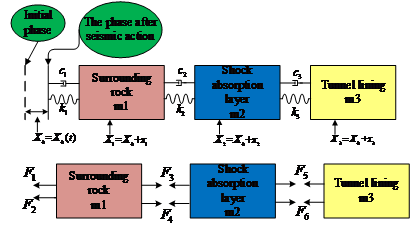Figure 2. The simplified mechanical model of the lining system

According to Figure 2, the displacement excitation by an earthquake on the surrounding rock is X0=X0(t), so the absolute displacement of each particle can be written as follows :

$X_{1}=X_{0}+x_{1}, X_{2}=X_{0}+x_{2}, X_{3}=X_{0}+x_{3}$     (1)

According to D’ Alembert’s theory, the motive equation of the tunnel structural system can be:

$[M]\{\ddot{x}\}+[C]\{\dot{x}\}+[K]\{x\}=\{P(t)\}$     (2)

where, $[M]$ is the whole mass matrix; $[C]$ is the whole damp matrix; $[K]$ is the whole stiffness matrix; $\{\ddot{x}\}$ is the systemic acceleration vector; $\{\dot{x}\}$ is the systemic velocity vector; $\{x\}$ is the systemic displacement vector; and $\{P(t)\}$ is the exciting force induced by an earthquake.

For formula $(2),$ in a complex domain, the ground motion caused by the displacement of the surrounding rock is $X_{0}(t)=$ $A e^{i w_{0} t},$ so $\ddot{X}_{0}(t)=-A w_{0}^{2} e^{i w_{0} t} .$ Assuming that $m_{1}=m, m_{2}=\alpha m,$ and $m_{3}=\beta m,$ and substituting these into $\{P(t)\}$ we can obtain:

$\{P(t)\}=-\ddot{X}_{0}\left\{\begin{array}{l}m_{1} \\ m_{2} \\ m_{3}\end{array}\right\}=\left\{\begin{array}{c}A w_{0}^{2} \\ \alpha A w_{0}^{2} \\ \beta A w_{0}^{2}\end{array}\right\}$

$m e^{i w_{0} t}=\left\{\begin{array}{l}P_{m 1} \\ P_{m 2} \\ P_{m 3}\end{array}\right\} e^{i w_{0} t}=\left\{P_{m}\right\} e^{i w_{0} t}$     (3)

where, $w_{0}$ is the frequency of earthquake excitation; and $\{P(t)\}=\left\{P_{m}\right\} e^{i w_{0} t}$ is the equivalent excitation induced by the earthquake.

Under the equivalent excitation induced by an earthquake, assume that the systematic steady-state forced response is:

$\{x(t)\}=\left\{\begin{array}{l}x_{1}(t) \\ x_{2}(t) \\ x_{3}(t)\end{array}\right\}=\left\{\begin{array}{l}A_{1} \\ A_{2} \\ A_{3}\end{array}\right\} e^{i w_{0} t}=\{A\} e^{i w_{0} t}$     (4)

Then, $\{\dot{x}(t)\}=i w_{0}\{A\} e^{i w_{0} t}$ and $\{\ddot{x}(t)\}=-w_{0}^{2}\{A\} e^{i w_{0} t}$, where $\{A\}=\left\{\begin{array}{l}A_{1} \\ A_{2} \\ A_{3}\end{array}\right\}$ is the complex amplitude. To solve it in the complex domain:

$\{A\}=\left\{\begin{array}{l}A_{1} \\ A_{2} \\ A_{3}\end{array}\right\}=\frac{A w_{0}^{2}}{\left[D\left(w_{0}\right)\right]}\left[\begin{array}{ccc}d_{22} d_{33}-d_{23}^{2} & -d_{12} d_{33} & d_{12} d_{23} \\ -d_{12} d_{33} & d_{11} d_{33} & -d_{11} d_{23} \\ d_{12} d_{23} & -d_{11} d_{23} & d_{11} d_{22}-d_{12}^{2}\end{array}\right]\left\{\begin{array}{l}m_{1} \\ m_{2} \\ m_{3}\end{array}\right\}=\frac{m A w_{0}^{2}}{\left\|D\left(w_{0}\right)\right\|} | \begin{array}{c}d_{22} d_{33}-d_{23}^{2}-\alpha d_{12} d_{33}+\beta d_{12} d_{23} \\ -d_{12} d_{33}+\alpha d_{11} d_{33}-\beta d_{11} d_{23} \\ d_{12} d_{23}-\alpha d_{11} d_{23}+\beta d_{11} d_{22}-\beta d_{12}^{2}\end{array}$     (5)

Assume that:

$H_{3}=\frac{\left|A_{3}\right|}{A}=\frac{w_{0}^{2} \sqrt{e^{2}+f^{2}}}{\sqrt{g^{2}+h^{2}}}$     (6)

and if $\eta_{i j}=\frac{m w_{i}^{2}}{k_{j}}, \xi_{i j}=\frac{c_{j}}{2 m w_{i}}(i, j=1,2,3), \mu=\frac{w_{0}}{w_{1}}, \gamma_{1}=\frac{k_{2}}{k_{1}}, \gamma_{2}=\frac{k_{2}}{k_{3}}, \mu_{1}=\frac{c_{2}}{c_{1}}, \mu_{2}=\frac{c_{2}}{c_{3}},$ then $\eta_{12}=\eta_{11} \frac{1}{\gamma_{1}}, \eta_{13}=\eta_{11} \frac{\gamma_{2}}{\gamma_{1}}$, $\xi_{12}=\mu_{1} \xi_{11}, \xi_{13}=\xi_{11} \frac{\mu_{1}}{\mu_{2}} .$ Substituting these into formula (6), we can obtain:

$H_{3}=\frac{\left|A_{3}\right|}{A}=\frac{\mu^{2} \sqrt{e^{12}+4 \mu f^{\prime 2}}}{\sqrt{g^{\prime 2}+h^{\prime 2}}}$     (7)

where, $H_{3}=\frac{\left|A_{3}\right|}{A}$ is defined as the transfer coefficient of deformation;

$e^{\prime}=\left\{\eta_{11}^{2} \mu^{2} \frac{\gamma_{2}}{\gamma_{1}^{2}}\left[\alpha \beta \mu^{2}-4 \xi_{11}^{2}\left[\beta \mu_{1}+(\alpha+\beta) \frac{\mu_{1}}{\mu_{2}}+(\alpha+\beta+1) \frac{\mu_{1}^{2}}{\mu_{2}}\right]\right]\right.$

$\left.-\eta_{11} \mu^{2}\left[\frac{\alpha \beta \gamma_{2}}{\gamma_{1}^{2}}+\frac{(\alpha+\beta)+\gamma_{2}(\beta+\alpha \beta)}{\gamma_{1}}\right]+\left[\frac{\beta \gamma_{2}+\alpha+\beta}{\gamma_{1}}+(\alpha+\beta+1)\right]\right\} \eta_{11}$     (8)

$f^{\prime}=\left\{\left[\left[\beta \mu_{1}+(\alpha+\beta) \frac{\mu_{1}}{\mu_{2}}\right] \frac{\gamma_{2}}{\gamma_{1}^{2}}+\left[\beta+(\alpha+\beta+1) \frac{\mu_{1}}{\mu_{2}}\right] \frac{\gamma_{2}}{\gamma_{1}}+\frac{(\alpha+\beta)+\mu_{1}(\alpha+\beta+1)}{\gamma_{1}}\right]\right.$

$\left.-\eta_{11} \mu^{2} \frac{\gamma_{2}}{\gamma_{1}^{2}}\left[\alpha \beta+\mu_{1}(\beta+\alpha \beta)+\frac{(\alpha+\beta) \mu_{1}}{\mu_{2}}\right]\right\} \xi_{11} \eta_{11}^{2}$     (9)

$g^{\prime}=\left\{4 \xi_{11}^{2} \mu^{2}\left[\frac{\mu_{1} \eta_{11}^{2}}{\gamma_{1}}\left(\beta \eta_{11} \frac{\gamma_{2}}{\gamma_{1}} \mu^{2}-1\right)+\frac{\mu_{1} \gamma_{2} \eta_{11}^{2}}{\mu_{2} \gamma_{1}}\left[(\alpha+\beta) \eta_{11} \frac{\mu^{2}}{\gamma_{1}}-1\right]\right.\right.$

$\left.\left.+\frac{\mu_{1}^{2} \gamma_{2} \eta_{11}^{2}}{\mu_{2} \gamma_{1}^{2}}\left[(\alpha+\beta+1) \eta_{11} \mu^{2}-1\right]\right]-\alpha \beta \frac{\gamma_{2} \eta_{11}^{3}}{\gamma_{1}^{2}}\left(\mu^{2}-1\right)\left(\mu^{2}-\frac{\eta_{2} \gamma_{1}}{\eta_{11}}\right)\left(\mu^{2}-\frac{\eta_{33} \gamma_{1}}{\eta_{11} \gamma_{2}}\right)\right\}$     (10)

$g^{\prime}=\left\{4 \xi_{11}^{2} \mu^{2}\left[\frac{\mu_{1} \eta_{11}^{2}}{\gamma_{1}}\left(\beta \eta_{11} \frac{\gamma_{2}}{\gamma_{1}} \mu^{2}-1\right)+\frac{\mu_{1} \gamma_{2} \eta_{11}^{2}}{\mu_{2} \gamma_{1}}\left[(\alpha+\beta) \eta_{1} \frac{\mu^{2}}{\gamma_{1}}-1\right]\right.\right.$

$\left.\left.+\frac{\mu_{1}^{2} \gamma_{2} \eta_{1}^{2}}{\mu_{2} \gamma_{1}^{2}}\left[(\alpha+\beta+1) \eta_{11} \mu^{2}-1\right]\right]-\alpha \beta \frac{\gamma_{2} \eta_{1}^{3}}{\gamma_{1}^{2}}\left(\mu^{2}-1\right)\left(\mu^{2}-\frac{\eta_{22} \gamma_{1}}{\eta_{11}}\right)\left(\mu^{2}-\frac{\eta_{33} \gamma_{1}}{\eta_{11} \gamma_{2}}\right)\right\}$     (11)

$h^{\prime}=\left\{\eta_{11}^{2}\left[\mu^{5}\left[\beta+(\beta+\alpha \beta) \mu_{1}+(\alpha+\beta) \frac{\mu_{1}}{\mu_{2}}\right]+4 \mu^{3} \xi_{11}^{2} \frac{\mu_{1}^{2}}{\mu_{2}}\right] \frac{\gamma_{2}}{\gamma_{1}^{2}}\right.$

$-\eta_{11} \mu^{3}\left[\left[\beta \mu_{1}+(\alpha+\beta) \frac{\mu_{1}}{\mu_{2}}\right] \frac{\gamma_{2}}{\gamma_{1}^{2}}+\left[\beta+(\alpha+\beta+1) \frac{\mu_{1}}{\mu_{2}}\right] \frac{\gamma_{2}}{\gamma_{1}}\right.$

$\left.\left.+\left[(\alpha+\beta)+(\alpha+\beta+1) \mu_{1}\right] \frac{1}{\gamma_{1}}\right]+\mu\left(1+\frac{\mu_{1}}{\gamma_{1}}+\frac{\mu_{1} \gamma_{2}}{\mu_{2} \gamma_{1}}\right)\right\} \xi_{11} \eta_{11}$     (12)

Defining $\lambda=\frac{m w^{2}}{k_{1}}$, then the characteristics matrix of the system under the condition without damping is:

$\left[D\left(w^{2}\right)\right]=[K]-w^{2}[M]=\alpha \beta \lambda^{3}\left[-\alpha \beta+\frac{\gamma_{1}^{2}}{\gamma_{2}} \alpha \beta+\gamma_{1} \beta+\frac{\gamma_{1}}{\gamma_{2}}(\alpha+\beta)\right] \lambda^{2}$

$+\left[\frac{\gamma_{1}}{\gamma_{2}}(\alpha+\beta)+\frac{\gamma_{1}^{2}}{\gamma_{2}}(\alpha-\beta+1)+\gamma_{1} \beta\right] \lambda-\frac{\gamma_{1}^{2}}{\gamma_{2}}=0$     (13)

If the system mass ratios $\alpha$ and $\beta$ and the stiffness ratios $\gamma_{1}$ and $\gamma_{2}$ are known, then the value of $\lambda$ can be obtained from the formula ( 13 ) and the previous formulas.

$\lambda_{1}=\eta_{11}, \frac{\lambda_{2}}{\lambda_{1}}=\frac{w_{2}^{2}}{w_{1}^{2}}=\frac{\eta_{22}}{\eta_{11}}, \gamma_{1} \frac{\lambda_{3}}{\lambda_{1}}=\frac{w_{3}^{2}}{w_{1}^{2}}=\frac{\eta_{33}}{\eta_{11}} \frac{\gamma_{1}}{\gamma_{2}}$     (14)

There are nine unknowns in the formula (14). If the system mass, stiffness, and damping can be determined, the variables $\alpha, \beta, \gamma_{1}, \gamma_{2}, \mu_{1}, \mu_{2},$ and $\lambda_{1}$  will be determined immediately.

3. The Damping Effect of Stiffness Change under the Condition of Different Frequency Ratios

The constraint relationship between the tunnel and the surrounding rock is complex. Their dynamic characteristics are more complicated under the influence of ground motion. To improve the effect of shock absorption, the damping material should have greater damping values. In this study, the variables $\xi_{11}=0.05, \alpha=0.6, \beta=1.4, r_{2}=0.05 \sim 1.5, k_{1}=4.5 \times 10^{9} \mathrm{Pa}$ and $k_{3}=3 \times 10^{10}$ Pa were determined, so $\gamma_{1}$ is calculated according to the stiffness of the surrounding rock. The frequency ratio $\mu$ is $0.05,0.1,0.5,1.0,2.0,3.0,4.0,6.0,8.0$ and $10.0 .$ The calculation results are shown in Figure 3.

As it is shown from Figure 3:

(1) When the frequency ratio $\mu$ is approximately $0.05-0.1$ the transfer coefficient of deformation first increases and then decreases with the increase in the stiffness ratio of the shock absorption layer and lining. Moreover, the value of the deformation transfer coefficient is less than $1,$ which indicates that the shock absorption layer can protect the lining well under the low-frequency vibration (the frequency ratio is less than $1 / 10$ ).

(2) When the frequency ratio is $0.1<\mu<2,$ the deformation transfer coefficient increases sharply and then gradually decreases, close to $1 .$ The peak deformation transfer coefficient decreases gradually with the increase in the frequency ratio, and the position of the peak changes with the increase in the ratio of frequency. This indicates that the vibration within this range of the frequency ratio $(0.1-2)$ can be reduced to a certain extent by a smaller stiffness ratio.

(3) When the frequency ratio $\mu>2,$ the position of the peak deformation transfer coefficient appears near to where the stiffness ratio is $1,$ and at the same time, the deformation transfer coefficients are less than 1 in this position. This indicates that the shock absorption layer (the frequency ratio is greater than 2 ) will not produce the ideal damping effect under high-frequency vibration. However, taking the shock absorption layer as the second liner (namely, double-lining), it may have a better protective effect on the tunnel lining.

## 31.png## 32.png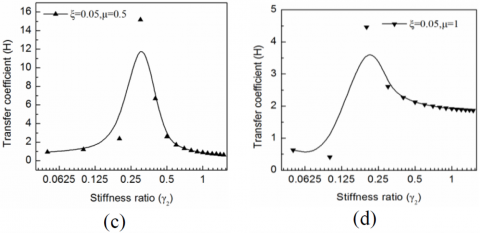## 33.png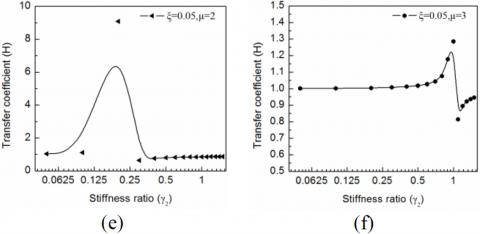## 34.png## 35.png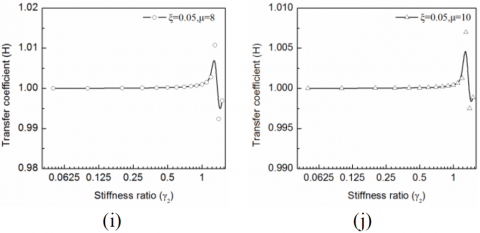Figure 3. The relationship between the transfer coefficient of deformation and the stiffness ratio $\gamma_{2}$

4. The Relationship between the Damping Ratio and the Damping Effect

The dynamic characteristics of the tunnel and the surrounding rock are influenced by earthquakes. The effect of damping should be considered in the study of the shock absorption of the tunnel.

In this study, it was determined that $\mu=0.05,$ the damping ratios $\xi_{11}$ are $0.05,0.10,0.50$ and $1.00,$ and the other parameters are the same. The calculation results are shown in Figure 4.

As it is shown from Figure 4:

The peak transfer coefficient declines gradually with the increase in the damping ratio when the frequency ratio $\mu=0.5$. This shows that under the same conditions, an increase in the damping ratio can effectively reduce the peak of the transfer coefficient, but it has little effect on the transfer coefficient outside of the peak.

## 4.png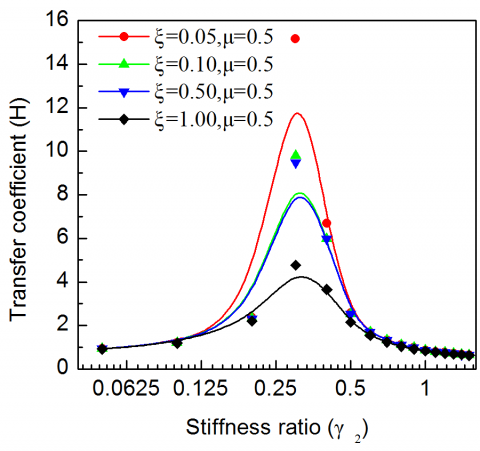Figure 4. The relationship between the transfer coefficient and the shock absorption layer and the lining stiffness ratio under different damping ratios

5. Numerical Calculation

5.1 Numerical examples

Taking a shallow-buried tunnel entrance section as an example, a $2 \mathrm{D}$ finite element model for a single-hole shallowburied tunnel model is built in MIDAS, as shown in Figure 5 . The width of the model is $\mathrm{B}=250 \mathrm{m},$ and the height of the model is $\mathrm{H}=90 \mathrm{m} .$ The top surface is assumed to be the ground. A simulation can be conducted for the tunnel and the first tunnel lining through the four point element (i.e., tetrahedron element) and the rectangular element for the second tunnel lining to present a dynamic finite element analysis. The MohrCoulomb criterion is adopted for the surrounding rock, and it has the Young's modulus of $\mathrm{E}_{0}=200 \mathrm{MPa}$, a Poisson's ratio of $v=0.32,$ a mass density of $\rho=1860 \mathrm{kg} / \mathrm{m}^{3},$ cohesion $C=0.5 \mathrm{MPa}$ and an interior frictional angle $\Phi=35^{\circ} .$ The shock absorption layer is assumed to be elastic, and it has the Young's modulus of $\mathrm{E}_{1}=3 \mathrm{GPa},$ a Poisson's ratio of $v=0.25$ and a mass density of $\rho=1110 \mathrm{kg} / \mathrm{m}^{3} .$ The second lining structure is assumed to be elastic, with a unit weight of $26 \mathrm{kN} / \mathrm{m}^{3},$ a Poisson's ratio of $0.15,$ and a Young's modulus of $\mathrm{E}=30 \mathrm{GPa}$. The finite element mesh partitioning strategy has great influence on the accuracy, convergence and efficiency of finite element calculation. In general, the optimal unit size of the finite element model not only meets the precision requirement of a dynamic solution but also satisfies computational efficiency. According to the research , the element size should meet the following conditions:

$\Delta x_{\max } \leq(1 / 6 \sim 1 / 8) \lambda_{\min }$     (15)

$\lambda_{\min }=v_{\min } / f_{\max }$     (16)

where, $v_{\min }$ is the minimum of the shear or compression wave velocity, and $f_{\max }$ is the highest vibration frequency of the input motion. For this study, $v_{\min }=200 \mathrm{m} / \mathrm{s}$ and $f_{\max }=50 \mathrm{Hz}$ Accordingly, the maximum size of element $\Delta x_{\max }$ should be less than approximately $0.5-0.67 \mathrm{m} .$ The mesh size of the $2 \mathrm{D}$ FEM model is defined as $0.5 \times 0.5 \mathrm{m},$ which satisfies the accuracy requirement.

5.2 Governing equation

According to D’ Alembert’s theory , the governing equation of the tunnel structural system can be:

$[M]\{\ddot{x}\}+[C]\{\dot{x}\}+[K]\{x\}=-[M]\left\{\ddot{x}_{g}\right\}$     (17)

where, $[M]$ is the whole mass matrix; $[C]$ is the whole damp matrix; $[K]$ is the whole stiffness matrix; $\{\ddot{x}\}$ is the systemic acceleration vector; $\{x\}$ is the systemic velocity vector; $\{x\}$ is the systemic displacement vector; and $\left\{\ddot{x}_{g}\right\}$ is the ground acceleration vector induced by the earthquake.

The Rayleigh damping matrix can be written as follows:

$C=\alpha M+\beta K$     (18)

where,

$\alpha=\zeta_{0} \cdot w_{0}, \beta=\zeta_{0} / w_{0}$     (19)

where, $\xi_{0}$ is the damping ratio, which is 0.05 for these calculations.

5.3 Calculating process

In the course of numerical calculation, the stiffness of the shock absorption layer is assumed to be constant. The second lining’s stiffness is taken as 0.5 E, 0.75 E, 1.0 E, 1.5 E, 2.0 E, 2.5 E and 3.0 E. Then, when the second lining stiffness is 1.0 E, the damping lay ness is 0.15 GPa, 0.45 GPa, 0.75 GPa, 1.5 GPa, 3 GPa and 4.5 GPa. The Mayazi Earthquake wave record in the Wenchuan Earthquake in 2008 is employed for the incident P waves. Various parameters of the tunnel system are shown in Table 1. The acceleration-time history of the record is plotted in Figure 6. The displacement, the maximum internal force and the stress of the lining crown, spandrel, abutment, and foundation of the support as well as the inverted arch are analysed through seismic wave input in the horizontal direction, with the damping ratio of the model as $\xi_{11}=0.05$ and the frequency ratio of seismic wave to the above system as $\mu=4$ The calculation results are shown in Figures 7-11.

## 5.png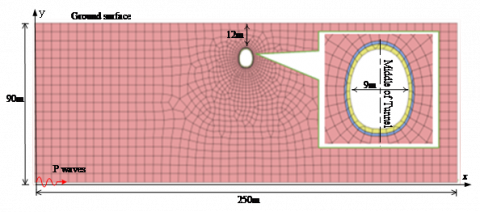Figure 5. Finite element mesh of shallow buried tunnel

## 6.pngFigure 6. Acceleration time-history number

Table 1. Mechanical parameters of the rock and tunnel lining

 Material type Specific gravity Young's modulus Poisson's ratio Cohesion Interior frictional angle kN/m3 E/GPa μ C/MPa φ/° Surrounding rock 22 4.5 0.32 0.5 35 Second lining 25 30 0.2 / /

## 7.png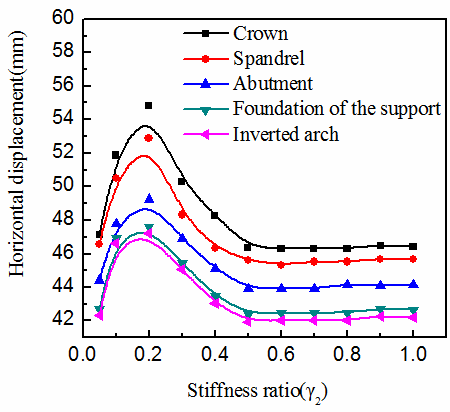Figure 7. The horizontal displacement curve of each characteristic point of the tunnel lining with the changing stiffness ratio

## 8.png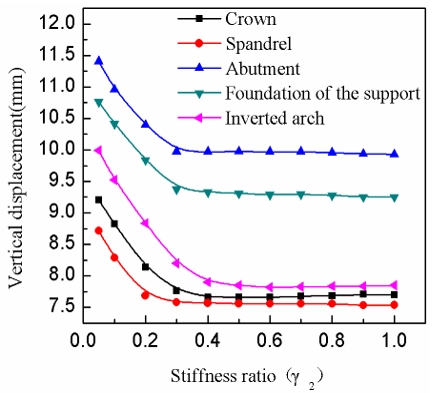Figure 8. The vertical displacement curve of each characteristic point of the tunnel lining with the changing stiffness ratio

## 9.pngFigure 9. The peak axial force curve of the tunnel lining with the changing stiffness ratio

## 10.png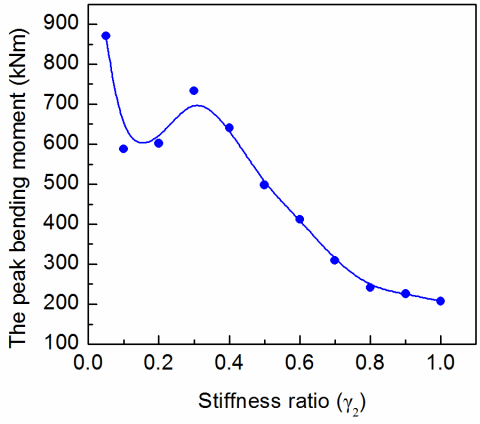Figure 10. The peak bending moment curve of the tunnel lining with the changing stiffness ratio

## 11.png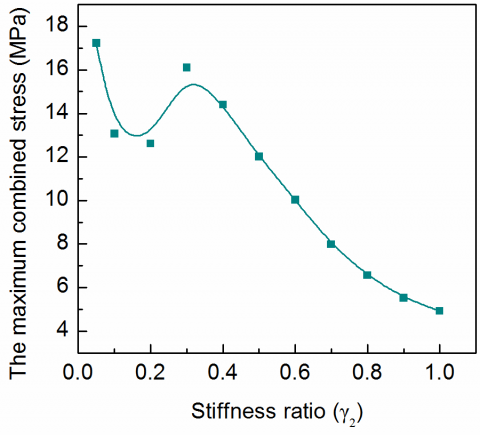Figure 11. The maximum combined stress curve of the tunnel lining with the changing stiffness ratio

As it is shown from Figures 7-11:

(1) The maximum horizontal displacement of each feature point of the tunnel lining is significantly greater than that of the vertical displacement under the conditions of seismic wave input along the horizontal direction.

(2) With the increase in the shock absorption layer and the stiffness ratio of the lining, the horizontal lateral displacement increases first and then decreases, and the vertical displacement decreases gradually; all displacements have multiple peaks. The displacement hardly changes with the increase in the stiffness ratio when the stiffness ratio is greater than 0.4.

(3) The axial force and the maximum axial stress of the lining increase first and then decrease with the increase in the shock absorption layer and the stiffness ratio of the lining; they display an approximately symmetrical distribution at both sides of 0.33.

(4) With the increase in the shock absorption layer and the lining stiffness ratio, the peak bending moment, which is the maximum combined stress of the lining, decreases gradually.

6. Conclusions

To reduce the seismic response of the tunnel lining, it is necessary to choose the proper stiffness ratio. A shock absorption layer is set between the tunnel lining and the surrounding rock, and the minor stiffness ratio between the shock absorption layer and the lining can effectively reduce the vibration response of the lining under low-frequency vibration. For high-frequency vibration, the minor stiffness ratio cannot reduce the vibration response of the lining, while when the stiffness ratio increases and approaches 1, the vibration response of the lining can be reduced. Through the numerical analysis under the condition of high-frequency vibration and when the stiffness ratio is reduced, the displacement response of the lining increases, and the axial force decreases; instead, the bending moment and bending stress increase. Therefore, double-lining can effectively protect the tunnel lining in areas with high seismic intensity.

Acknowledgment

The paper is funded by Gansu Provincial Science and Technology Program (18YF1GA055).

References

 Li, T.B. (2008). Failure characteristics and influence factor analysis of mountain tunnels at epicenter zones of great Wenchuan earthquake. Journal of Engineering Geology, 16: 742-750. https://doi.org/10.3969/j.issn.1004-9665.2008.06.003

 Arlikatti, S., Huang, S.K., Yu, C.H., Hua, C.L. (2019). ‘Drop, cover and hold on’ or ‘Triangle of life’ attributes of information sources influencing earthquake protective actions. International Journal of Safety and Security Engineering, 9(3): 213-224. https://doi.org/10.2495/SAFE-V9-N3-213-224

 Ma, A.C., Wang, S.L. (2019). Semi-active control of continuous girder bridges considering the coupling effect of earthquake and hydrodynamic pressure. International Journal of Heat and Technology, 37(4): 1187-1194. https://doi.org/10.18280/ijht.370429

 Roy, N., Sarkar, R. (2017). A Review of seismic damage of mountain tunnels and probable failure mechanisms. Geotechnical and Geological Engineering, 35: 1-28. https://doi.org/10.1007/s10706-016-0091-x

 Zhang, X.P., Jiang, Y.J., Sugimoto, S. (2018). Seismic damage assessment of mountain tunnel: A case study on the Tawara Yama tunnel due to the 2016 Kumamoto Earthquake. Tunneling and Underground Space Technology, 71: 138-148. https://doi.org/10.1016/j.tust.2017.07.019

 Hu, S.G., Wu, X.F., Xi, Z.F. (2012). Impact of direct tunneling current on CMOS logic circuit. International Journal of Digital Content Technology & its Applic, 6: 231-238. https://doi.org/CNKI:SUN:DQDZ.0.2011-06-017

 Yu, J., Liu, G.Y., Cai, Y.Y., Zhou, J.F., Liu, S.Y., Tu, B.X. (2020). Time-Dependent deformation mechanism for swelling soft-rock tunnels in coal mines and its mathematical deduction. International Journal of Geomechanics, 20(3): 04019186. https://doi.org/10.1061/(ASCE)GM.1943-5622.0001594

 Dikmen, S.U. (2016). Response of Marmaray submerged tunnel during 2014 northern Aegean earthquake (Mw=6.9). Soil Dynamics and Earthquake Engineering, 90: 15-31. https://doi.org/10.1016/j.soildyn.2016.08.006

 Halber, A., Chakravarty, D. (2018). Investigation of wireless tracking performance in the tunnel-like environment with particle filter. Mathematical Modelling of Engineering Problems, 5(2): 93-101 https://doi.org/10.18280/mmep.050206.

 Okamoto, S., Tamura, C. (1972). Behaviour of subaqueous tunnels during earthquakes. Earthquake Engineering & Structural Dynamics, 1(3): 253-266. https://doi.org/10.1002/eqe.4290010306

 Goto, Y., Matsuda, Y., Ejiri, J., Ito, K. (1988). Influence of distance between juxtaposed shield tunnels on their seismic responses. In: Proceedings of the 9th World Conference on Earthquake Engineering, pp. 569-574.

 Xu, H., Li, T., Xia, L., Zhao, J.X., Wang, D. (2016). Shaking table tests on seismic measures of a model mountain tunnel. Tunnelling and Underground Space Technology, 60(Complete): 197-209. https://doi.org/10.1016/j.tust.2016.09.004

 Chen, G., Wang, Z., Zuo, X., Du, X., Gao, H. (2013). Shaking table test on the seismic failure characteristics of a subway station structure on liquefiable ground. Earthquake Engineering and Structural Dynamics, 42(10): 1489-1507. https://doi.org/10.1002/eqe.2283

 Tsinidis, G., Rovithis, E., Pitilakis, K., Chazelas, J.L. (2016). Seismic response of box-type tunnels in soft soil: Experimental and numerical investigation. Tunnelling and Underground Space Technology, 59: 199-214. https://doi.org/10.1016/j.tust.2016.07.008

 Kontoe, S., Avgerinos, V., Potts, D.M. (2014). Numerical validation of analytical solutions and their use for equivalent-linear seismic analysis of circular tunnels. Soil Dynamics and Earthquake Engineering, 66: 206-219. https://doi.org/10.1016/j.soildyn.2014.07.004

 Lanzano, G., Bilotta, E., Russo, G., Silvestri, F. (2015). Experimental and numerical study on circular tunnels under seismic loading. European Journal of Environmental and Civil Engineering, 19(5): 539-563. https://doi.org/10.1080/19648189.2014.893211

 Wang, M.N., Cui, G.Y. (2010). Establishment of tunnel damping model and research on damping effect with model test in highly seismic area. Rock and Soil Mechanics, 6: 1884-1890. https://doi.org/NKI:SUN:YTLX.0.2010-06-035

 Cao, G.A. (1997). Study on underground structural stochastic seismic response and extreme value analysis. Beijing Jiaotong University Doctor Degree Dissertation.

 Shen, G.Q., Meng, X.J., Wang, Y.M., Niu, X.M., Sun, Z.T. (2004). Random seismic inversion and its application in Chengbei No. 35 Well-zone. Oil Geophysical Prospecting, 39(1): 75-81. https://doi.org/10.3321/j.issn:1000-7210.2004.01.015

 Yan, S.H., Gao, F., Gao, B. (2005). Analysis of dynamic aseismic reliability of underground structures. Journal of the China Railway Society, 26: 96-100. https://doi.org/10.3321/j.issn:1000-6915.2005.01.012

 Yan, S.H., Liang, B., Gao, B. (2005). Dynamic analysis of longitudinal aseismic reliability of underground structures. Chinese Journal of Rock Mechanics and Engineering, 24(1): 71-76. https://doi.org/10.3321/j.issn:1000-6915.2005.01.012

 Du, X.L. (2008). Theories and Methods of Wave Motion for Engineering. Science Press, Beijing, Science Press, 282.

 Zhang, Y., Fu, X., Sheng, Q. (2014). Modification of the discontinuous deformation analysis method and its application to seismic response analysis of large underground caverns. Tunnelling and Underground Space Technology, 40: 241-250. https://doi.org/10.1016/j.tust.2013.10.012